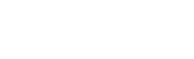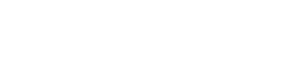# Introduction to Speed, Distance and Time | Cambridge IGCSE Maths[Please watch the video attached at the end of this blog for a visual explanation of speed, time, and distance]

In today’s article, we will be discussing the important concepts of speed, distance, and time that you can find to be tested in almost every Mathematics paper.

## Speed

To start things off, we will first learn the meaning of speed. Speed is the rate at which an object moves/ travels. Out of this definition, we can very easily come up with the formula to determine speed, when both time and distance are given.Example: How fast is a car traveling if it travels 400 meters in 20 seconds?

We use the formula Speed = Distance ÷ Time and substitute the given values in the necessary places.

400 ÷ 20 = 20

Therefore the speed is 20 m/s, which you can also write as 20 m/s-1.

## Time

In Physics, and sometimes in Mathematics, time can be defined as change or the interval in which change happens. If we apply it to the formula that we have above, we can slightly rearrange the formula by swapping speed and time.

Then the new formula in order to find time would be:Example: If a car travels a distance of 216 kilometers with a speed of 72 km/h, what is the time taken for the car to travel?

Here too we use the formula, substitute the values and find the answer.

Time = Distance ÷ Speed

Time  = 216 km ÷ 72 km/h  3 hours

• Time is given in hours because the entire question is in the format of kilometers and hours. However, even if one value was in meters and seconds, then there will be another calculation involved in order to turn kilometers into meters and hours into seconds, or vice versa.

## Distance

We can rearrange this formula once more to find out the distance traveled.Example: If a car travels at 9 km/h for two hours what is the distance traveled by car?

Distance = Speed ✕ Time

Distance  9 km/h ✕ 2h = 18 km/h

Helpful points for practicing speed, time, and distance for your upcoming Cambridge IGCSE Mathematics exams.

Mathematics is a subject that relies heavily on how well you prepare in advance for your exams. To make things easier, here are a few pointers that may help you get ready for your upcoming Cambridge IGCSE Mathematics exams.

Study one formula that has speed, time, and distance in them and you can answer any question since you just need to swap them around depending on the question you get.

Practice sample questions – Practise, practice, practice. Questions on speed, time and distance come in all shapes and sizes. They can sometimes be quite tricky, so do attempt all the questions you can get your hands on before exams. Our question banks have questions of different difficulty levels that you can practice before your actual exams.

Practice past paper questions to familiarise yourself with the format of the questions and paper.

Ask for help – You are never too late to ask for assistance if you’re struggling. Reach out to us at Tutopiya to find the right tutor to help you pass your exams with flying colors!

Watch the video below for a visual explanation of speed, distance, and time and attempt the quiz to challenge yourself!